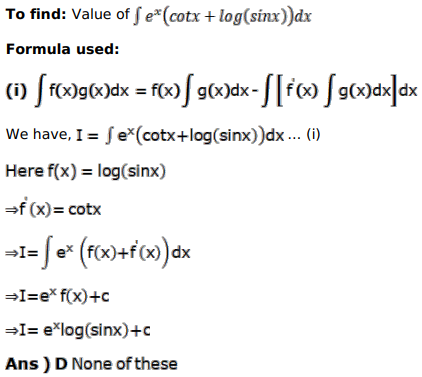# Mark against the correct answer in each of the following:

Question:

Mark $(\sqrt{)}$ against the correct answer in each of the following:

$\int e^{x}(\cot x+\log \sin x) d x=?$

A. $e^{x} \log (\sec x+\tan x)+C$

B. $e^{x} \sec x+C$

C. $e^{x} \log \tan x+C$

D. none of these

Solution: